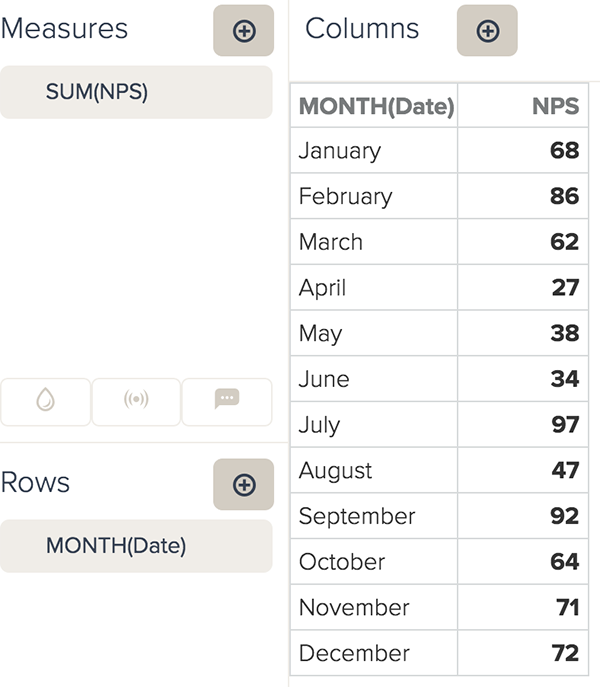# Recipe: Analyzing Net Promoter Score calculations

Follow

When you are looking to analyze your NPS, your dataset will normally contain numeric values for the score. With the variety of metric aggregators, it is very easy to create calculations for analyzing the average, minimum, or maximum of your score. Alternatively, you can use your score as an attribute to view how your results are divided amongst your different groups.

This tip contains the following sections:

Skill level : Beginner

Time : 10 minutes

Ingredients :
• One calculated group attribute
• Three calculated metrics

## Calculating NPS using score as an attribute

When you are calculating your NPS analysis, you can use score as an attribute to visualize how results are divided between the highest and lowest scores. If you use score as an attribute, you will need to create different calculated metrics for your NPS than when using score as a metric. If you do not want to use score as an attribute, you can follow the steps in Calculating NPS using score as a metric .

There are three steps required for analyzing your NPS metric and using your score as an attribute:

### Editing the score element in the Data source Builder

Initially your score element will be added to your dataset as a metric. If you want to use your score as an attribute, you will need to edit the element in the Schema step of the Data source Builder.

1. Select your data source and enter the required credentials (see Adding connections ).
2. In the Schema step, select your score element. The example dataset below uses Mark as the score element.
3. Click Copy Left (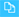) to duplicate your score metric as an attribute. After you import your data, Metrics will be added to the end of your score metric.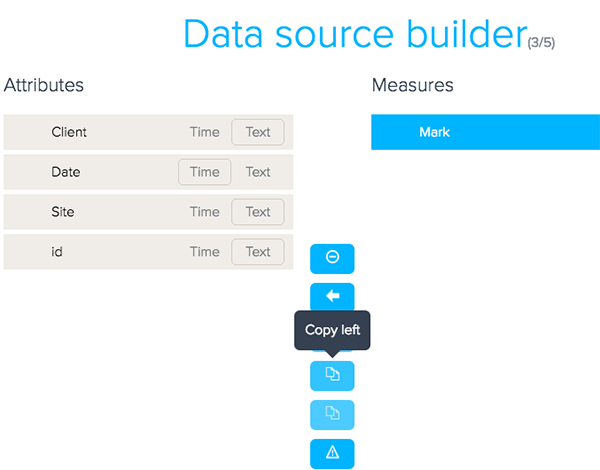4. When you are finished establishing the connection, click Save . You will be automatically redirected to the Query Builder.

Before you can create calculations, you first need to create a calculated group attribute. The group attribute will divide your score into promoters, detractors, and passives. The groups will be used as the basis for your calculations.
1. In the Calculation engine menu, select Group .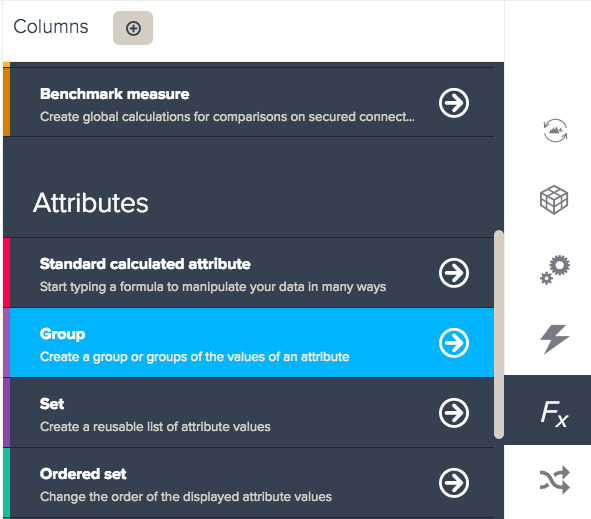2. Name your group attribute Groups.
3. Select your score attribute from the Computed from drop-down list.
4. Select your highest values, then click the + button to add a new group.
5. Click on the group name, then rename the group Promoters.
6. Select your lowest values, then click the + button to add a new group.
7. Rename the group Detractors.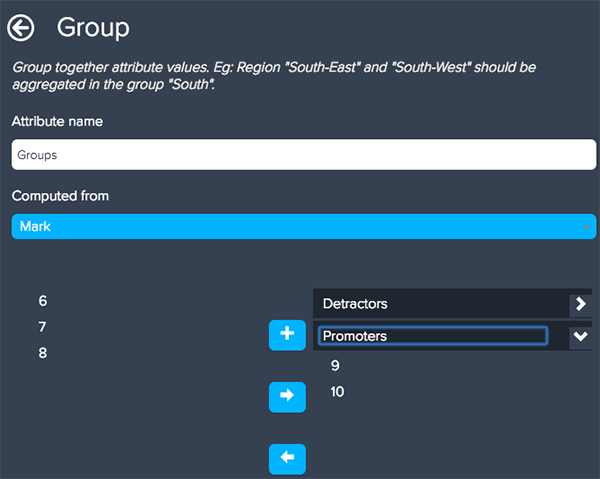8. From the drop-down list underneath your groups, select Put left values into another group . The remaining values will represent your passive scores.
9. Name the remaining values group Passives.10. Click Save when you are finished.

To create your NPS calculation, you will need to create three different calculated metrics.
1. In the Calculation engine menu, select Standard calculated metric .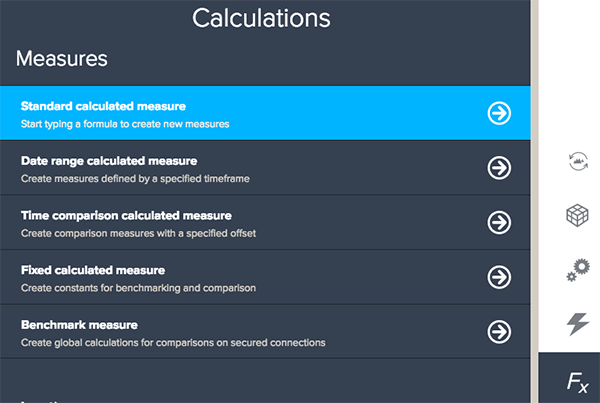2. Type the following formulas for each new calculated metric. Make sure you click Save after each metric. If you do not click Save , the metric will not be created.
• Promoters:
`IF ([Groups]="Promoters") THEN SUM(Responses) ENDIF`
• Detractors:
`IF ([Groups]="Detractors") THEN SUM(Responses) ENDIF`
• NPS:
`(SUM(Promoters)/SUM(Responses)*100)-(SUM(Detractors)/SUM(Responses)*100)`

The image below is an example of a report displaying the percentage representation of each group.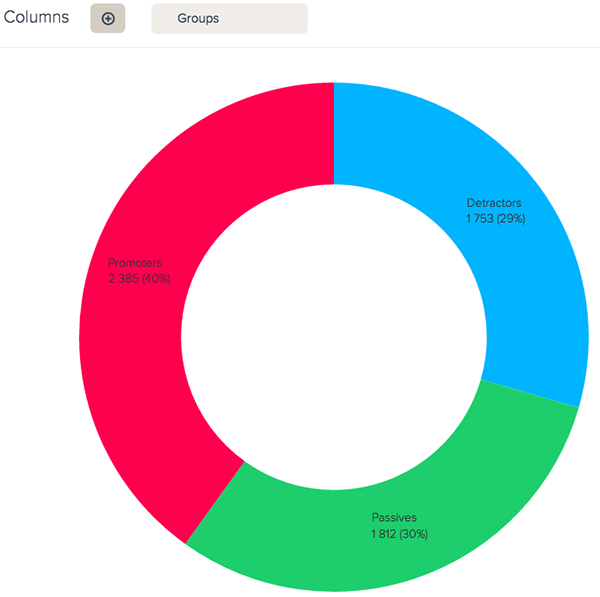## Calculating NPS using score as a metric

If you do not want to divide your results by score, you can create calculations using the original score metric. You do not need to edit your score metric to create these calculations.

Note: On larger datasets calculating NPS without groups might take longer to process. If there are too many results to process in-memory, you might have to delegate to a database.
To create calculations based on the original NPS metric
1. In the Calculation engine menu, select Standard calculated metric .
2. Type the following formulas for each new calculated metric:
• Promoters:
`IF (SUM(Mark)<7 THEN SUM(Responses) ENDIF`
• Detractors:
`IF (SUM(Mark)>8) THEN SUM(Responses) ENDIF`
• NPS:
Note: This formula requires the SUMX aggregator rather than the SUM aggregator used in the calculations above.
```(SUMX(Promoters metric)/SUM(Responses)*100) - (SUMX(Detractors
metric)/SUM(Responses)*100)```